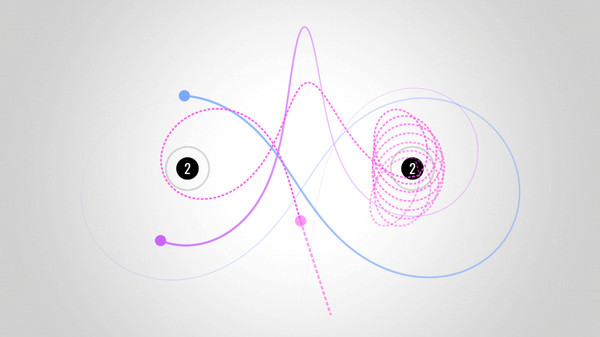# 2D trajectory prediction around planets

I’ve created a game where the player fires a projectile between planets trying to hit a target. The planets then apply their gravitational forces to the projectile. All of this is working correctly. What I now want is to use a LineRenderer to give a prediction of where the projectile will fly. This is where my math skills fail me.

My code/scene

All projectiles originate from one location and always at the same initial speed. The only variable that changes is the direction.
When a projectile is fired I apply a force to the rigidbody

``````rb.AddForce(origin.up * VELOCITY)
``````

As soon as the projectile is spawned all gravitational entities in the scene starts pulling on it.

``````float forceMagnitude = (projectileMass * planetGravity) / Mathf.Pow(distance, 2);
Vector2 force = direction.normalized * forceMagnitude;
``````

Thoughts

I’ve experimented somewhat trying to solve this and have managed to get a line which loops around the planets. However it doesn’t match the path the projectile flies. So something is off. I think my calculation around applying gravity and how to simulate the initial velocity.

My goal is to have something similar to the game Orbit (Steam)Do you guys have any insight in how I can tackle this? I think I just need a nudge in the right direction.

Of course… Solved it almost exactly 20 minutes after asking the question…
So for anyone else trying to solve the same thing, now or in the future, here is my solution:

``````void FixedUpdate()
{
Vector2 velocity = origin.up * 100 * Time.fixedDeltaTime;
lineRenderer.positionCount = segmentCount;
lineRenderer.SetPosition(0, origin.position);

float dt = Time.fixedDeltaTime;

for (int i = 1; i < segmentCount; i++)
{
Vector2 previous = _lineRenderer.GetPosition(i - 1);
velocity += CalculateGravity(previous) * dt;
lineRenderer.SetPosition(i, previous + velocity * dt);
}
}
``````